# Constructing a Binary Tree from Its Preorder & Inorder Traversal

In this article we are going to see, how to construct a binary tree from its preorder & inorder traversals?
Submitted by Radib Kar, on August 04, 2020

Prerequisite:

In this article, we see how to construct a tree from its preorder & inorder traversal?

In the inorder traversal, we first store the left subtree then the root and finally the right subtree.

Where in the preorder traversal, we first store the root and then the left subtree and finally the right subtree.

Let's just assume we are given with the preorder traversal only. Can we build the tree only from preorder traversal?

Of course no. Because, though we find the root as the starting element in the preorder element, we can't find its subtrees from the rest of the traversal.

For example, say the preorder traversal is: 1 2 3 4 6 5

We know that root of the tree is 1

But we have no idea about which part is left subtree and which part is right subtree. But for sure [2, 3, 4, 5, 6] is the traversal for the subtrees and may be starting few elements or no elements fall in left subtree and rest in right subtree. So, we can't draw the partition b/w the subtrees. For this purpose, the inorder traversal helps. Because, in order traversal, if we find the root, then it's guaranteed that whichever lies before the root falls in its left subtree & whichever lies after the root falls in its right subtree.

Like the inorder traversal of the tree which has the previous preorder traversal is: 2 1 6 4 3 5

Here, we know that tree root is 1(we came to know from the preorder traversal of course). So it's guaranteed that  falls in its left subtree & [6, 4, 3, 5] falls in its right tree.

So till now what we have is shown below: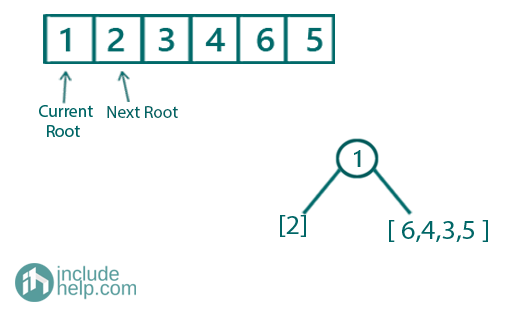So the root is formed as a node. We know what part of array its left subtree and what part of array is its right subtree.

Now we will build the subtrees recursively. To build the left subtree we will do exactly same. We will have the root which is just the next element in the preorder traversal (so root is 2 now).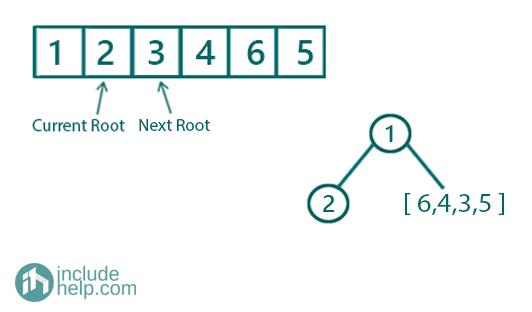On the other hand,

To build the right subtree we will do exactly same. We will have the root which is just the next element in the preorder traversal (so root is 3 now). Similarly we will search 3 in the inorder traversal.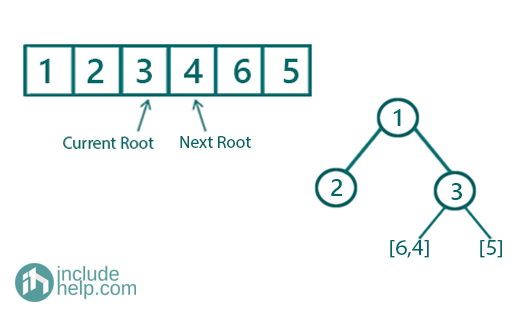Further recursions: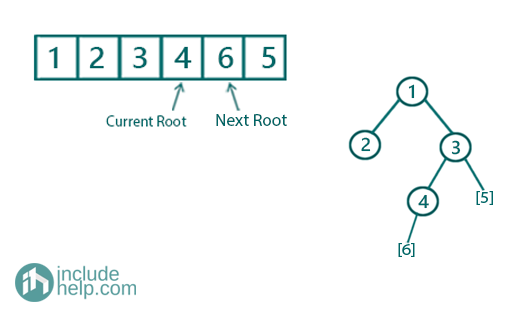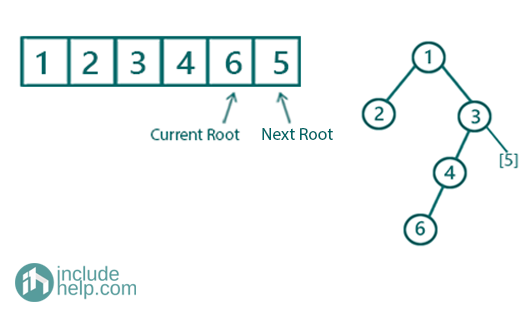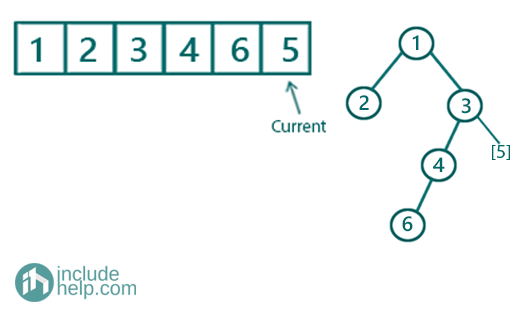Final built tree is: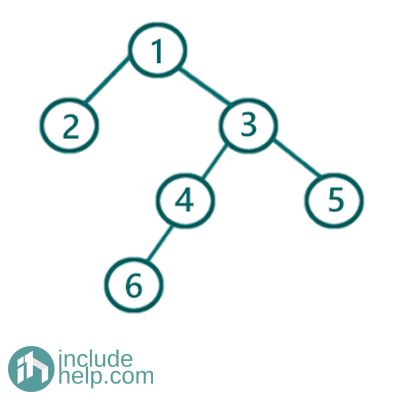C++ Implementation:

```#include <bits/stdc++.h>
using namespace std;

class TreeNode {
public:
int val;
TreeNode* left;
TreeNode* right;

TreeNode(int v)
{
val = v;
left = NULL;
right = NULL;
}
};

//searching the root value in the
//inorder traversal stored vector
int search(vector<int> a, int k)
{
for (int i = 0; i < a.size(); i++)
if (a[i] == k)
return i;

return -1;
}

TreeNode* buildTreeRecur(vector<int>& preorder, vector<int>& inorder, int& index, int left, int right)
{
if (index == preorder.size())
return NULL;
//base case to return child of leaf nodes NULL
if (left > right)
return NULL;
/*
preorder[index] is the root
search that in the inorder vector,
say position found is in_index
then [left,k-1] will have the left subtree
for the current root
[k+1,right] will have traversal for right subtree
for the current rooot
recursively build the subtrees
*/
int in_index = search(inorder, preorder[index]);
int k = preorder[index];
index++;
//root created
TreeNode* root = new TreeNode(k);
//recursively building the subtree
root->left = buildTreeRecur(preorder, inorder, index, left, in_index - 1);
root->right = buildTreeRecur(preorder, inorder, index, in_index + 1, right);
return root;
}

TreeNode* buildTree(vector<int>& preorder, vector<int>& inorder)
{
int index = 0;
int n = preorder.size();
return buildTreeRecur(preorder, inorder, index, 0, n - 1);
}

void levelOrder(TreeNode* root)
{
queue<TreeNode*> q;

q.push(root);
q.push(NULL);

int count = 0;
while (!q.empty()) {
TreeNode* temp = q.front();
q.pop();
if (!temp) {
cout << "\nEnd of level: " << count << endl;
count++;
if (!q.empty()) {
q.push(NULL);
}
}
else {
cout << temp->val << " ";
if (temp->left)
q.push(temp->left);
if (temp->right)
q.push(temp->right);
}
}
}

int main()
{
//preorder traversal is stored in vector preorder
//inorder traversal is stored in vector inorder
vector<int> preorder{ 1, 2, 3, 4, 6, 5 };
vector<int> inorder{ 2, 1, 6, 4, 3, 5 };

TreeNode* root = buildTree(preorder, inorder);

cout << "Level order traversal of the tree built is:\n";
levelOrder(root);

return 0;
}
```

Output:

```Level order traversal of the tree built is:
1
End of level: 0
2 3
End of level: 1
4 5
End of level: 2
6
End of level: 3
```